# 【三维路径规划】基于matlab RRT算法无人机三维路径规划【含Matlab源码 1363期】

## 一、RRT算法简介

0 引言 随着现代技术的发展，飞行器种类不断变多，应用也日趋专一化、完善化，如专门用作植保的大疆PS-X625无人机，用作街景拍摄与监控巡察的宝鸡行翼航空科技的X8无人机，以及用作水下救援的白鲨MIX水下无人机等，决定飞行器性能主要是内部的飞控系统和外部的路径规划问题。就路径问题而言，在具体实施任务时仅靠操作员手中的遥控器控制无人飞行器执行相应的工作，可能会对操作员心理以及技术提出极高的要求，为了避免个人操作失误，进而造成飞行器损坏的危险，一种解决问题的方法就是对飞行器进行航迹规划。 飞行器的测量精度，航迹路径的合理规划，飞行器工作时的稳定性、安全性等这些变化对飞行器的综合控制系统要求越来越高。无人机航路规划是为了保证无人机完成特定的飞行任务，并且能够在完成任务的过程中躲避各种障碍、威胁区域而设计出最优航迹路线的问题。常见的航迹规划算法如图1所示。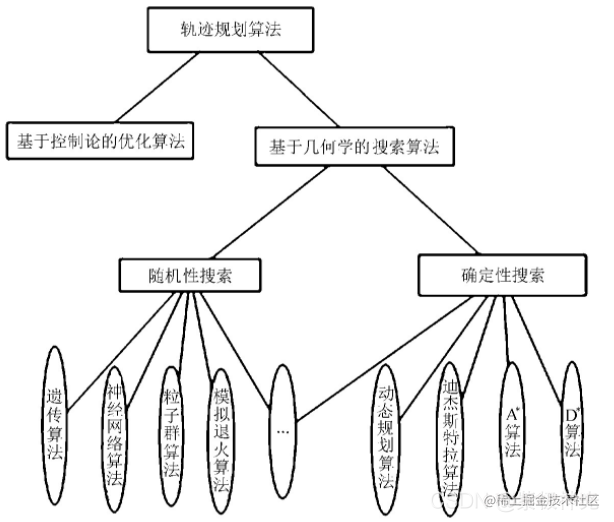图1 常见路径规划算法 文中主要对无人机巡航阶段的航迹规划进行研究，假设无人机在飞行中维持高度与速度不变，那么航迹规划成为一个二维平面的规划问题。在航迹规划算法中,A算法计算简单，容易实现。在改进A算法基础上，提出一种新的、易于理解的改进A算法的无人机航迹规划方法。传统A算法将规划区域栅格化，节点扩展只限于栅格线的交叉点，在栅格线的交叉点与交叉点之间往往存在一定角度的两个运动方向。将存在角度的两段路径无限放大、细化，然后分别用两段上的相应路径规划点作为切点，找到相对应的组成内切圆的圆心，然后作弧，并求出相对应的两切点之间的弧所对应的圆心角，根据下式计算出弧线的长度式中：R———内切圆的半径； α———切点之间弧线对应的圆心角。

## 二、RRT算法简介

1 RRT定义 RRT（Rapidly-Exploring Random Tree）算法是一种基于采样的路径规划算法，常用于移动机器人路径规划，适合解决高维空间和复杂约束下的路径规划问题。基本思想是以产生随机点的方式通过一个步长向目标点搜索前进，有效躲避障碍物，避免路径陷入局部极小值，收敛速度快。本文通过matlab实现RRT算法，解决二维平面的路径规划问题。 2 地图 为了方便算法的实现，使用离散量来表达环境地图。其中，数值0表示无障碍物的空区域，数值1表示该区域有障碍物。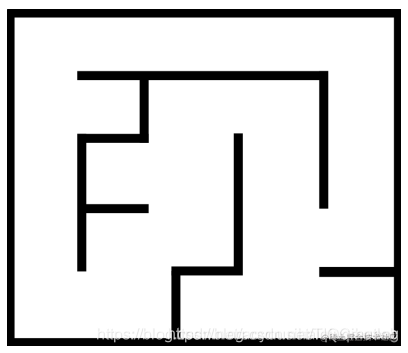RRT算法中搜索到的顶点坐标为连续点，在地图中产生随机点，算法将通过连续的点构建树。此过程中，对树枝和顶点进行检测，检测顶点所处位置是否是空区域。下载附录中.dat文件，绘制地图。

``````colormap=[1 1 1; 0 0 0; 1 0 0; 0 1 0; 0 0 1];
imshow(uint8(map),colormap)

note:数据中的列为x轴，行为y轴

3 RRT算法原理 通过matlab程序构建从起始位置到目标位置的树，并生成连接两个点的路径。使用一颗中心点在起始点的树，而不是两颗树（一个中心点在起始位置，一个中心点在目标位置）。 编写一个matlab函数，输入和输出有相同的形式。

``````function [vertices, edges, path] = rrt(map, q_start, q_goal, k, delta_q, p)

4 障碍物检测 检测树枝（即q_near和q_new之间的edge）是否处于自由空间，可以使用增量法或者等分法，示意图如下(假设两点之间有10个点，左图为为增量检测法，右图为等分法，从示意图中可以看出使用等分法检测次数更少)：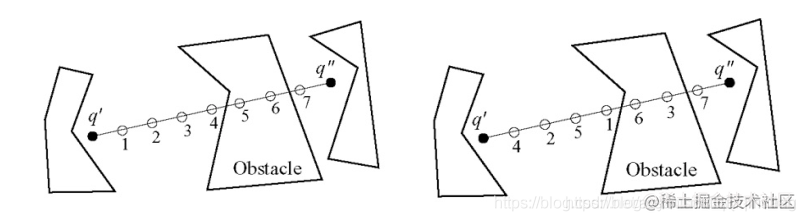在本文中，使用k=10000，delta_q=50,p=0.3, 我们将获得如下结果：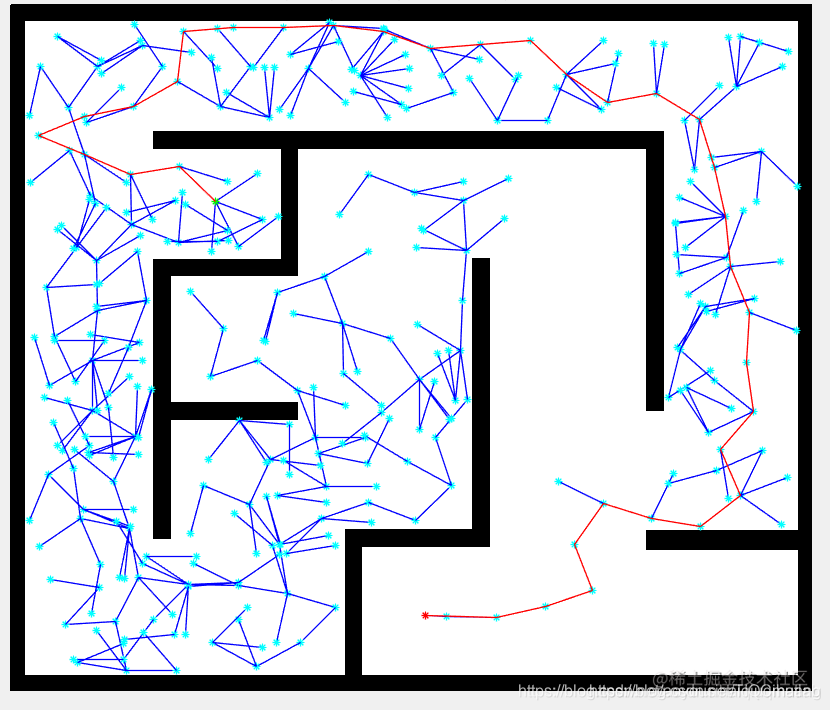5 路径平滑处理 完成基本的RRT算法之后，我们获得了一条从起点到终点的路径，现在对这条路径进行平滑和降噪处理，处理完成之后，我们将得到一条更短的路径。 采用贪心算法： 连接q_start和q_goal,如果检测到两个点之间有障碍物，则将q_goal替换为前一个点，直到两个点能连接上（中间无障碍物）为止。一旦q_goal被连接上， 在matlab中定义平滑函数：

``````function [path_smooth] = smooth(map, path, vertices, delta)

## 三、部分源代码

``````function c;
clc
clear all
close all
%map1 随机地表。
% a=10;
% b=0.2;
% c=0.1;
% d=0.6;
% e=1;
% f=0.1;
% g=0.1;
% for x=1:80
%     for y=1:80
% Z1=sin(y+a)+b*sin(x)+cos(d*(x^2+y^2)^(1/2))+e*cos(y)+f*sin(f*(x^2+y^2)^(1/2))+g*cos(y);
% % Z1=SquareDiamond(6,2,8);
%  figure(1);
%  surf(Z1); %画出三维曲面
% %map2 山峰图
tic;
h=[20,35,25,38,20,25];
x0=[10,40,45,60,20,20];
y0=[10,25,50,30,45,10];
xi=[5.5,8,5,4.5,5.5,3.5];
yi=[5,7,6,5.5,6,4.5];
Z2=CeatHill(6,h,x0,y0,xi,yi,80);
figure(2);
surf(Z2); %画出三维曲面
%map3 合成图
%  Z3=max(Z1,Z2);
%  figure(3);
%  surf(Z3); %画出三维曲面
segmentLength =5;
start_node =  [5,70,5,0,0,0];
end_node   =[80,30,10,1,0,0];
hold on
plot3(start_node(:,1),start_node(:,2),start_node(:,3),'r*');
plot3(end_node(:,1),end_node(:,2),end_node(:,3),'r*');
tree = start_node;
if ( (norm(start_node(1:3)-end_node(1:3))<segmentLength )...
&(collision(start_node,end_node)==0) )
path = [start_node; end_node];
else
numPaths = 0;
while numPaths<1,
[tree,flag] = extendTree(tree,end_node,segmentLength,Z2);
numPaths = numPaths + flag;
end
end
path = findMinimumPath(tree);
plot3(path(:,1),path(:,2),path(:,3),'r');
toc;
function [data]=CeatHill(N,h,x0,y0,xi,yi,num)
x=1:1:num;y=1:1:num;
for m=1:num
for n=1:num
Sum=0;
for k=1:N
s=h(k)*exp(-((x(m)-x0(k))/xi(k))^2-((y(n)-y0(k))/yi(k))^2);
Sum=Sum+s;
end
data(m,n)=Sum;
end
end

function collision_flag = collision(node, parent,Z2,high);
collision_flag = 0;

x0=[10,40,45,60,20,20];
y0=[10,25,50,30,45,10];
xi=[5.5,8,5,4.5,5.5,3.5];
yi=[5,7,6,5.5,6,4.5];
Z1=Z2;
% for i=1:80
%     for j=1:80
%         if(Z1(j,i)>high)
%             Z1(j,i)=10000;
%         end
%     end
% end
if ((node(1)>80)...
| (node(1)<0)...
| (node(2)>80)...
| (node(2)<0))
collision_flag = 1;
else
for sigma = 0:0.1:1,
p = sigma*node(1:3) + (1-sigma)*parent(1:3);
Sum1=0;
for k=1:6

Sum1=Sum1+s;
end
if(p(3)<Sum1)
collision_flag = 1;
end
% [line,row]=find(Z1==10000);
% ct=length(line);
% for tt=1:ct
%     B=floor(p(1));
%     C=floor(p(2));
% if (B==line(tt,1)&C==row(tt,1))
%         collision_flag = 1;
% end
% end

end
end
function [new_tree,flag,high] = extendTree(tree,end_node,segmentLength,Z2);
flag1 = 0;
qet=1;
while flag1==0,
% select a random point
if(qet==0)
randomPoint = [...
80*rand,...
80*rand,...
80*rand];
else
randomPoint = [...
end_node(:,1),...
end_node(:,2),...
end_node(:,3)];
end
% find leaf on node that is closest to randomPoint
%0.45*sqrt(tree(:,1:2)-ones(size(tree,1),1)*randomPoint)
tmp = sqrt(tree(:,1:3)-ones(size(tree,1),1)*end_node(:,1:3));
[dist,idx] = min(diag(tmp*tmp'));

agl=acos((dot(A,B)/(norm(A)*norm(B))))*180/pi;
if (agl>80)
continue;
end
cost= tree(idx,4) + segmentLength;
new_point = (randomPoint-tree(idx,1:3));
new_point = tree(idx,1:3)+new_point/norm(new_point)*segmentLength;
if(qet==1)
high=new_point(:,3);
end
if(tree(idx,3)==end_node(:,3))
new_point(:,3)=end_node(:,3);
end
if(tree(idx,3)>end_node(:,3))
new_point(:,3)=tree(idx,3)-new_point(:,3)/norm(new_point)*segmentLength;
end
new_node = [new_point, 0, cost, idx];
%     hold on
if collision(new_node, tree(idx,:),Z2,high)==0,
new_tree = [tree; new_node];
%  plot3(new_point(:,1),new_point(:,2),new_point(:,3),'r*');
flag1=1;
else
qet=0;
flag1=0;
end
end
% check to see if new node connects directly to end_node
if ( (norm(new_node(1:3)-end_node(1:3))<segmentLength )...
&(collision(new_node,end_node,Z2,high)==0) )
flag = 1;
new_tree(end,4)=1;  % mark node as connecting to end. end没找到

function path = findMinimumPath(tree);
connectingNodes = [];
for i=1:size(tree,1),
if tree(i,4)==1,
connectingNodes = [connectingNodes; tree(i,:)];
end
end
% find minimum cost last node
[tmp,idx] = min(connectingNodes(:,5));

% construct lowest cost path
path = [connectingNodes(idx,:)];
parent_node = connectingNodes(idx,6);

while parent_node>1,
path = [tree(parent_node,:); path];
parent_node = tree(parent_node,6);

end

## 四、运行结果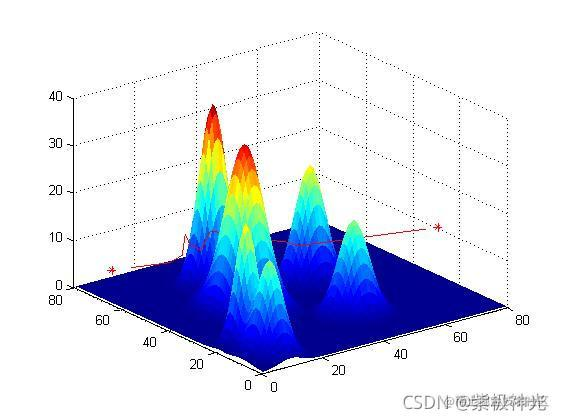## 五、matlab版本及参考文献

1 matlab版本 2014a

2 参考文献  包子阳,余继周,杨杉.智能优化算法及其MATLAB实例（第2版）[M].电子工业出版社，2016. 张岩,吴水根.MATLAB优化算法源代码[M].清华大学出版社，2017. RRT路径规划算法